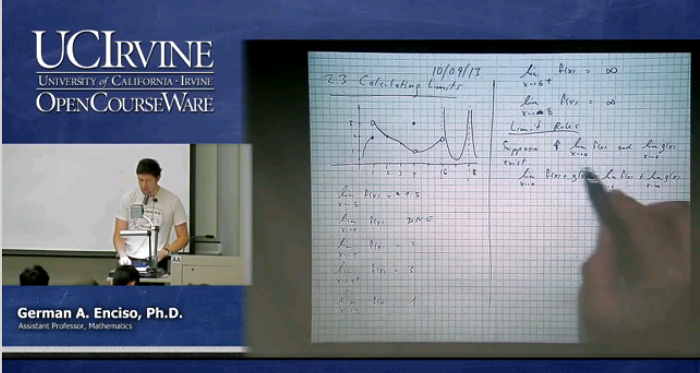# Home

## Math 2A. Lec. 06. Calculus: Calculating Limits (English)

Recorded: October 9, 2013

Lecture 6: Calculating Limits

Course Description: UCI Math 2A is the first quarter in Single-Variable Calculus and covers the following topics: Introduction to derivatives, calculation of derivatives of algebraic and trigonometric functions; applications including curve sketching, related rates, and optimization; exponential and logarithm functions.

Required attribution: Enciso, German A. Math 2A (UCI Open: University of California, Irvine), http://open.uci.edu/courses/math_2a_calculus_i.html. [Access date]. License: Creative Commons Attribution-ShareAlike 3.0 United States License.Author:
German Andres Enciso Ruiz
Title:
Assistant Professor
Department:
Mathematics